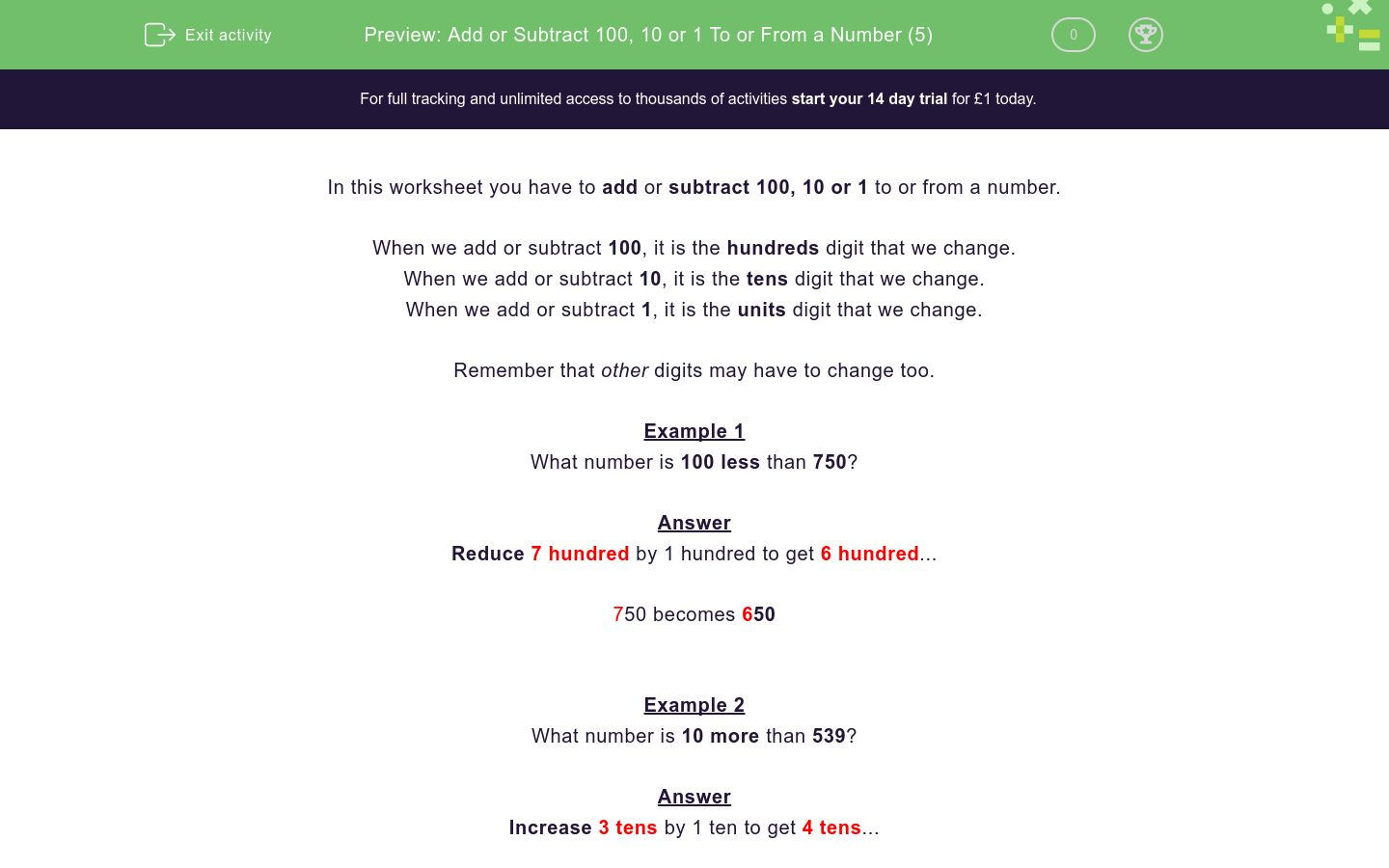# Add or Subtract 100, 10 or 1 To or From a Number (5)

In this worksheet, students add or subtract 100, 10 or 1 to or from a given number.Key stage:  KS 2

Curriculum topic:   Number: Number and Place Value

Curriculum subtopic:   Find 10 or 100 More or Less than a Given Number

Difficulty level:### QUESTION 1 of 10

In this worksheet you have to add or subtract 100, 10 or 1 to or from a number.

When we add or subtract 100, it is the hundreds digit that we change.

When we add or subtract 10, it is the tens digit that we change.

When we add or subtract 1, it is the units digit that we change.

Remember that other digits may have to change too.

Example 1

What number is 100 less than 750?

Reduce 7 hundred by 1 hundred to get 6 hundred...

750 becomes 650

Example 2

What number is 10 more than 539?

Increase 3 tens by 1 ten to get 4 tens...

539 becomes 549

Example 3

What number is 1 less than 539?

Reduce 9 units by 1 unit to get 8 units...

539 becomes 538

Example 4

What number is 10 less than 405?

Here we have to be careful, because we can't reduce the tens digit by 1.

So include the hundreds digit.

Think of it as reducing 40 tens by 1 ten to get 39 tens.

405 becomes 395

What number is 10 less than 217?

What number is 100 less than 362?

What number is 100 more than 50?

What number is 10 more than 436?

What number is 1 more than 259?

What number is 100 more than 400?

What number is 1 less than 770?

What number is 100 less than 263?

What number is 10 less than 304?

What number is 100 less than 171?

• Question 1

What number is 10 less than 217?

207
EDDIE SAYS
Reduce 1 ten by 1 ten to get 0 tens...
217 becomes 207
• Question 2

What number is 100 less than 362?

262
EDDIE SAYS
Reduce 3 hundred by 1 hundred to get 2 hundred...
362 becomes 262
• Question 3

What number is 100 more than 50?

150
EDDIE SAYS
Increase 0 hundreds by 1 hundred to get 1 hundred...
050 becomes 150
• Question 4

What number is 10 more than 436?

446
EDDIE SAYS
Increase 3 tens by 1 ten to get 4 tens...
436 becomes 446
• Question 5

What number is 1 more than 259?

260
EDDIE SAYS
Increase 59 units by 1 unit to get 60 units...
259 becomes 260
• Question 6

What number is 100 more than 400?

500
EDDIE SAYS
Increase 4 hundred by 1 hundred to get 5 hundred...
400 becomes 500
• Question 7

What number is 1 less than 770?

769
EDDIE SAYS
Reduce 70 units by 1 unit to get 69 units...
770 becomes 769
• Question 8

What number is 100 less than 263?

163
EDDIE SAYS
Reduce 2 hundred by 1 hundred to get 1 hundred...
263 becomes 163
• Question 9

What number is 10 less than 304?

294
EDDIE SAYS
Reduce 30 tens by 1 ten to get 29 tens...
304 becomes 294
• Question 10

What number is 100 less than 171?

71
EDDIE SAYS
Reduce 1 hundred by 1 hundred to get 0 hundreds...
171 becomes 071 which we write as 71
---- OR ----

Sign up for a £1 trial so you can track and measure your child's progress on this activity.

### What is EdPlace?

We're your National Curriculum aligned online education content provider helping each child succeed in English, maths and science from year 1 to GCSE. With an EdPlace account you’ll be able to track and measure progress, helping each child achieve their best. We build confidence and attainment by personalising each child’s learning at a level that suits them.

Get started